# PSAT Math : How to find the equation of a perpendicular line

## Example Questions

### Example Question #11 : How To Find The Equation Of A Perpendicular Line

Solve the system of equations for the point of intersection.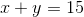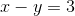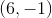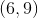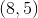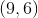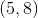Explanation:

First one needs to use one of the two equations to substitute one of the unknowns.

From the second equation we can derive that y = x – 3.

Then we substitute what we got into the first equation which gives us: x + x – 3 = 15.

Next we solve for x, so 2x = 18 and x = 9.

x – y = 3, so = 6.

These two lines will intersect at the point (9,6).

### Example Question #11 : How To Find The Equation Of A Perpendicular Line

Line A is perpendicular to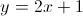and passes the point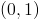. Find the-intercept of line A.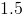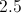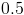Explanation:

We are given an equation of a line and told that line A is perpendicular to it.  The slope of the given line is 2.  Therefore, the slope of line A must be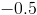, since perpendicular lines have slopes that are negative reciprocals of each other.

The equation for line A will therefore take the form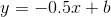, where b is the y-intercept.

Since we are told that it crosses, we can plug in the point and solve for c: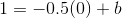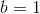Then the equation becomes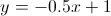.

To find the x-intercept, plug in 0 for y and solve for x: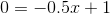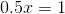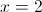### Example Question #13 : How To Find The Equation Of A Perpendicular Line

What line is perpendicular to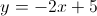through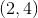?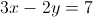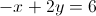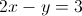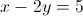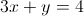Explanation:

The slope of the given line is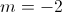, and the slope of the perpendicular line is its negative reciprocal,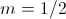.  We take the new slope and the given point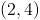and plug them into the slope-intercept form of a line,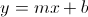.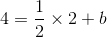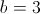Thus, the perpendicular line has the equation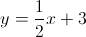, or in standard form,.

### Example Question #14 : How To Find The Equation Of A Perpendicular Line

In the xy-plane, the equation of the line n is –x+8y=17. If the line m is perpendicular to line n, what is a possible equation of line m?

y= -8x + 5

y= 8x-17

y= -1/8x + 5

x= -8y + (17/8)

y= -8x + 5

Explanation:

We start by add x to the other side of the equation to get the y by itself, giving us 8y =17 + x. We then divide both sides by 8, giving us y= 17/8 + 1/8x. Since we are looking for the equation of a perpendicular line, we know the slope (the coefficient in front of x) will be the opposite reciprocal of the slope of our line, giving us y= -8x + 5 as the answer.

### Example Question #1 : How To Find The Equation Of A Perpendicular Line

What line is perpendicular to x + 3y = 6 and travels through point (1,5)?

y = –1/3x – 4

y = 3x + 2

y = 6x – 3

y = 2x + 1

y = 2/3x + 6

y = 3x + 2

Explanation:

Convert the equation to slope intercept form to get y = –1/3x + 2.  The old slope is –1/3 and the new slope is 3.  Perpendicular slopes must be opposite reciprocals of each other:  m1 * m2 = –1

With the new slope, use the slope intercept form and the point to calculate the intercept: y = mx + b or 5 = 3(1) + b, so b = 2

So y = 3x + 2

### Example Question #1 : How To Find The Equation Of A Perpendicular Line

What line is perpendicular to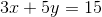and passes through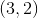?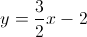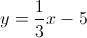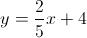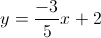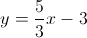Explanation:

Convert the given equation to slope-intercept form.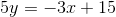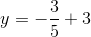The slope of this line is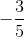. The slope of the line perpendicular to this one will have a slope equal to the negative reciprocal.

The perpendicular slope is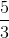.

Plug the new slope and the given point into the slope-intercept form to find the y-intercept.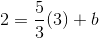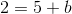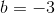So the equation of the perpendicular line is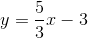.

### Example Question #3 : How To Find The Equation Of A Perpendicular Line

What is the equation of a line that runs perpendicular to the line 2x + = 5 and passes through the point (2,7)?

2x – y = 6

x/2 + y = 6

x/2 + y = 5

x/2 – y = 6

2x + y = 7

x/2 + y = 6

Explanation:

First, put the equation of the line given into slope-intercept form by solving for y. You get y = -2x +5, so the slope is –2. Perpendicular lines have opposite-reciprocal slopes, so the slope of the line we want to find is 1/2. Plugging in the point given into the equation y = 1/2x + b and solving for b, we get b = 6. Thus, the equation of the line is y = ½x + 6. Rearranged, it is –x/2 + y = 6.

### Example Question #4 : How To Find The Equation Of A Perpendicular Line

Line m passes through the points (1, 4) and (5, 2). If line p is perpendicular to m, then which of the following could represent the equation for p?

x  y = 3

4x  3y = 4

2x  y = 3

3x + 2y = 4

2x + y = 3

2x  y = 3

Explanation:

The slope of m is equal to   y2-y1/x2-x1  =  2-4/5-1 -1/2

Since line p is perpendicular to line m, this means that the products of the slopes of p and m must be 1:

(slope of p) * (-1/2) = -1

Slope of p = 2

So we must choose the equation that has a slope of 2. If we rewrite the equations in point-slope form (y = mx + b), we see that the equation 2x  y = 3 could be written as y = 2x – 3. This means that the slope of the line 2x – y =3 would be 2, so it could be the equation of line p. The answer is 2x – y = 3.

### Example Question #5 : How To Find The Equation Of A Perpendicular Line

What is the equation for the line that is perpendicular to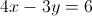through point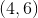?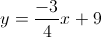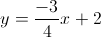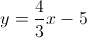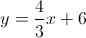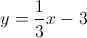Explanation:

Perpendicular slopes are opposite reciprocals.

The given slope is found by converting the equation to the slope-intercept form.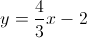The slope of the given line is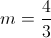and the perpendicular slope is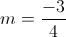.

We can use the given point and the new slope to find the perpendicular equation. Plug in the slope and the given coordinates to solve for the y-intercept.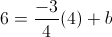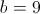Using this y-intercept in slope-intercept form, we get out final equation:.

### Example Question #1 : How To Find The Equation Of A Perpendicular Line

Which line below is perpendicular to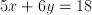?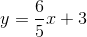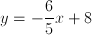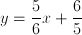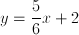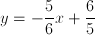Explanation:

The definition of a perpendicular line is one that has a negative, reciprocal slope to another.

For this particular problem, we must first manipulate our initial equation into a more easily recognizable and useful form: slope-intercept form or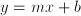.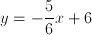According to ourformula, our slope for the original line is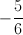. We are looking for an answer that has a perpendicular slope, or an opposite reciprocal. The opposite reciprocal ofis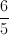. Flip the original and multiply it by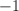Our answer will have a slope of. Search the answer choices forin the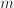position of theequation.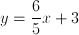is our answer.

(As an aside, the negative reciprocal of 4 is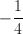. Place the whole number over one and then flip/negate. This does not apply to the above problem, but should be understood to tackle certain permutations of this problem type where the original slope is an integer.)